Publicité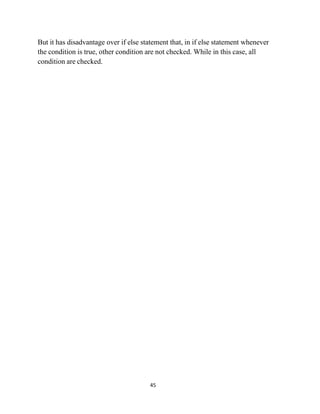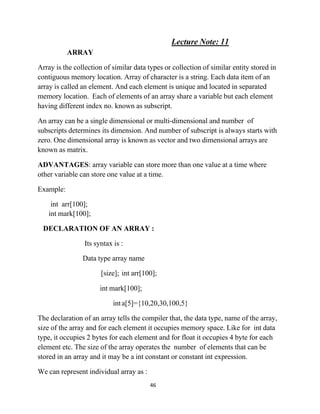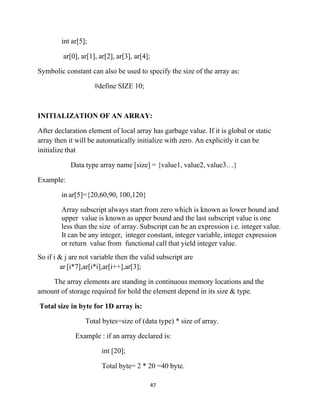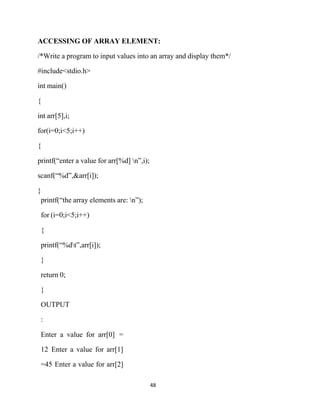Publicité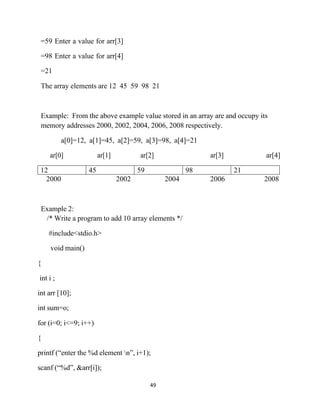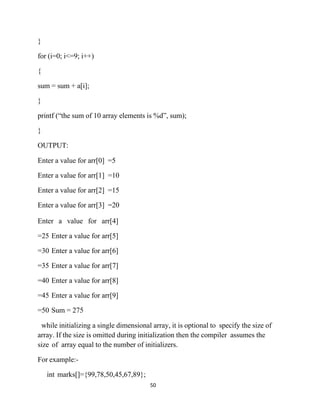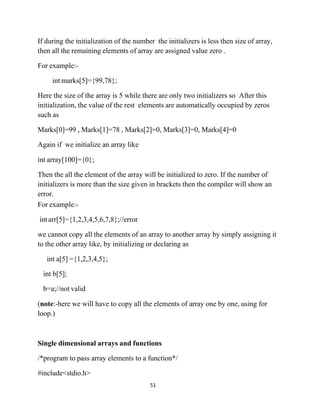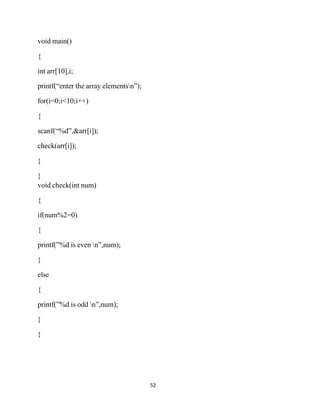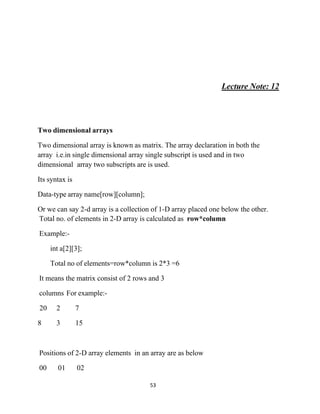Publicité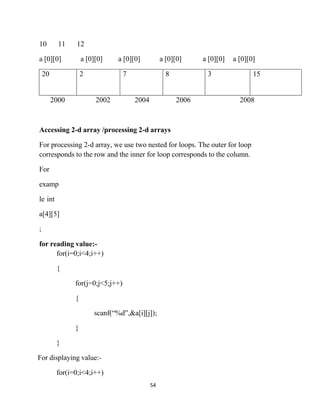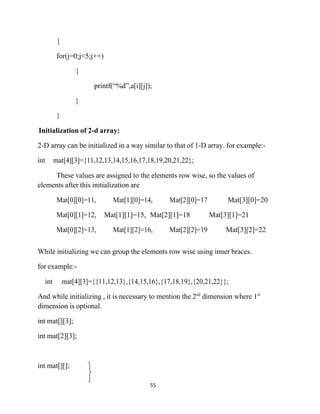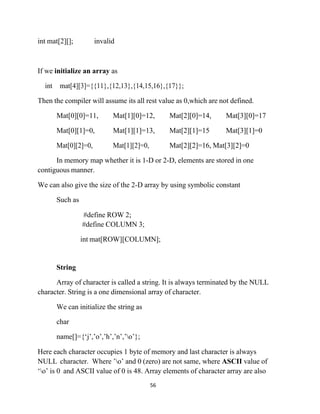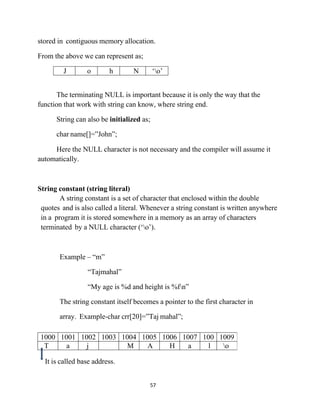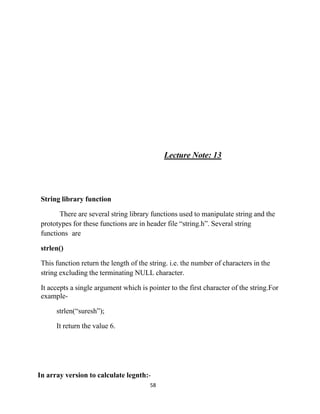Publicité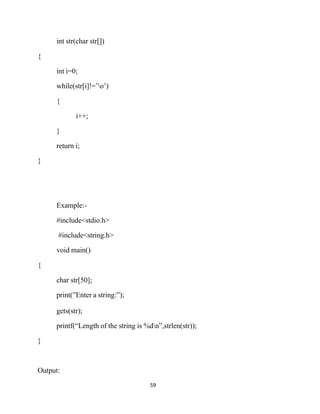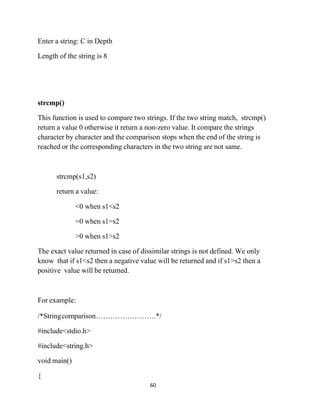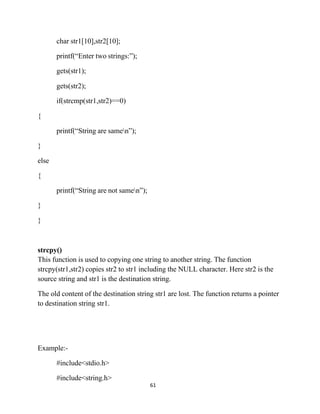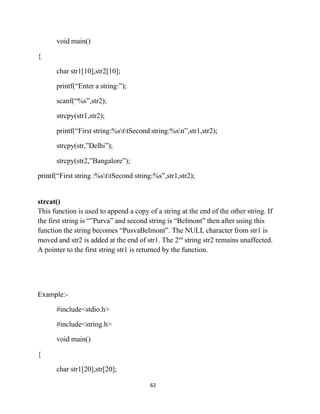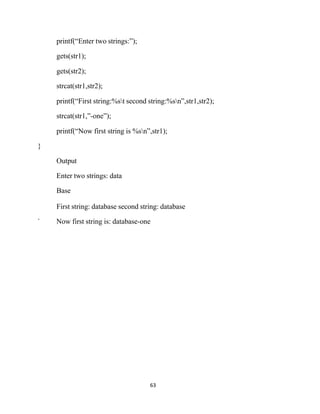Prochain SlideShare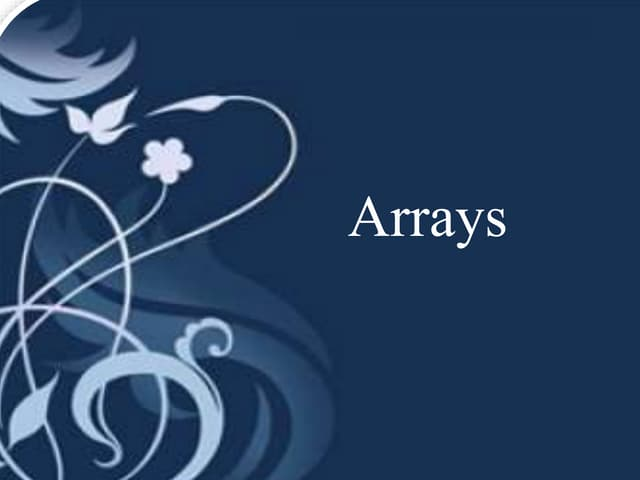Arrays in java
Chargement dans ... 3
1 sur 19
Publicité

### Array&amp;string

1. 45   But it has disadvantage over if else statement that, in if else statement whenever the condition is true, other condition are not checked. While in this case, all condition are checked.
2. 46   Lecture Note: 11 ARRAY Array is the collection of similar data types or collection of similar entity stored in contiguous memory location. Array of character is a string. Each data item of an array is called an element. And each element is unique and located in separated memory location. Each of elements of an array share a variable but each element having different index no. known as subscript. An array can be a single dimensional or multi-dimensional and number of subscripts determines its dimension. And number of subscript is always starts with zero. One dimensional array is known as vector and two dimensional arrays are known as matrix. ADVANTAGES: array variable can store more than one value at a time where other variable can store one value at a time. Example: int arr; int mark; DECLARATION OF AN ARRAY : Its syntax is : Data type array name [size]; int arr; int mark; inta={10,20,30,100,5} The declaration of an array tells the compiler that, the data type, name of the array, size of the array and for each element it occupies memory space. Like for int data type, it occupies 2 bytes for each element and for float it occupies 4 byte for each element etc. The size of the array operates the number of elements that can be stored in an array and it may be a int constant or constant int expression. We can represent individual array as :
3. 47   int ar; ar, ar, ar, ar, ar; Symbolic constant can also be used to specify the size of the array as: #define SIZE 10; INITIALIZATION OF AN ARRAY: After declaration element of local array has garbage value. If it is global or static array then it will be automatically initialize with zero. An explicitly it can be initialize that Data type array name [size] = {value1, value2, value3…} Example: in ar={20,60,90, 100,120} Array subscript always start from zero which is known as lower bound and upper value is known as upper bound and the last subscript value is one less than the size of array. Subscript can be an expression i.e. integer value. It can be any integer, integer constant, integer variable, integer expression or return value from functional call that yield integer value. So if i & j are not variable then the valid subscript are ar [i*7],ar[i*i],ar[i++],ar; The array elements are standing in continuous memory locations and the amount of storage required for hold the element depend in its size & type. Total size in byte for 1D array is: Total bytes=size of (data type) * size of array. Example : if an array declared is: int ; Total byte= 2 * 20 =40 byte.
4. 48   ACCESSING OF ARRAY ELEMENT: /*Write a program to input values into an array and display them*/ #include<stdio.h> int main() { int arr,i; for(i=0;i<5;i++) { printf(“enter a value for arr[%d] n”,i); scanf(“%d”,&arr[i]); } printf(“the array elements are: n”); for (i=0;i<5;i++) { printf(“%dt”,arr[i]); } return 0; } OUTPUT : Enter a value for arr = 12 Enter a value for arr =45 Enter a value for arr
5. 49   =59 Enter a value for arr =98 Enter a value for arr =21 The array elements are 12 45 59 98 21 Example: From the above example value stored in an array are and occupy its memory addresses 2000, 2002, 2004, 2006, 2008 respectively. a=12, a=45, a=59, a=98, a=21 ar ar ar ar ar 12 45 59 98 21 2000 2002 2004 2006 2008 Example 2: /* Write a program to add 10 array elements */ #include<stdio.h> void main() { int i ; int arr ; int sum=o; for (i=0; i<=9; i++) { printf (“enter the %d element n”, i+1); scanf (“%d”, &arr[i]);
6. 50   } for (i=0; i<=9; i++) { sum = sum + a[i]; } printf (“the sum of 10 array elements is %d”, sum); } OUTPUT: Enter a value for arr =5 Enter a value for arr =10 Enter a value for arr =15 Enter a value for arr =20 Enter a value for arr =25 Enter a value for arr =30 Enter a value for arr =35 Enter a value for arr =40 Enter a value for arr =45 Enter a value for arr =50 Sum = 275 while initializing a single dimensional array, it is optional to specify the size of array. If the size is omitted during initialization then the compiler assumes the size of array equal to the number of initializers. For example:- int marks[]={99,78,50,45,67,89};
7. 51   If during the initialization of the number the initializers is less then size of array, then all the remaining elements of array are assigned value zero . For example:- int marks={99,78}; Here the size of the array is 5 while there are only two initializers so After this initialization, the value of the rest elements are automatically occupied by zeros such as Marks=99 , Marks=78 , Marks=0, Marks=0, Marks=0 Again if we initialize an array like int array={0}; Then the all the element of the array will be initialized to zero. If the number of initializers is more than the size given in brackets then the compiler will show an error. For example:- intarr={1,2,3,4,5,6,7,8};//error we cannot copy all the elements of an array to another array by simply assigning it to the other array like, by initializing or declaring as int a ={1,2,3,4,5}; int b; b=a;//not valid (note:-here we will have to copy all the elements of array one by one, using for loop.) Single dimensional arrays and functions /*program to pass array elements to a function*/ #include<stdio.h>
8. 52   void main() { int arr,i; printf(“enter the array elementsn”); for(i=0;i<10;i++) { scanf(“%d”,&arr[i]); check(arr[i]); } } void check(int num) { if(num%2=0) { printf(”%d is even n”,num); } else { printf(”%d is odd n”,num); } }
9. 53   Lecture Note: 12 Two dimensional arrays Two dimensional array is known as matrix. The array declaration in both the array i.e.in single dimensional array single subscript is used and in two dimensional array two subscripts are is used. Its syntax is Data-type array name[row][column]; Or we can say 2-d array is a collection of 1-D array placed one below the other. Total no. of elements in 2-D array is calculated as row*column Example:- int a; Total no of elements=row*column is 2*3 =6 It means the matrix consist of 2 rows and 3 columns For example:- Positions of 2-D array elements in an array are as below 00 01 02 20 2 7 8 3 15
10. 54   10 11 12 a  a  a  a  a  a  20 2 7 8 3 15 2000 2002 2004 2006 2008 Accessing 2-d array /processing 2-d arrays For processing 2-d array, we use two nested for loops. The outer for loop corresponds to the row and the inner for loop corresponds to the column. For examp le int a ; for reading value:- for(i=0;i<4;i++) { for(j=0;j<5;j++) { scanf(“%d”,&a[i][j]); } } For displaying value:- for(i=0;i<4;i++)
11. 55   { for(j=0;j<5;j++) { printf(“%d”,a[i][j]); } } Initialization of 2-d array: 2-D array can be initialized in a way similar to that of 1-D array. for example:- int mat={11,12,13,14,15,16,17,18,19,20,21,22}; These values are assigned to the elements row wise, so the values of elements after this initialization are Mat=11, Mat=14, Mat=17 Mat=20 Mat=12, Mat=15, Mat=18 Mat=21 Mat=13, Mat=16, Mat=19 Mat=22 While initializing we can group the elements row wise using inner braces. for example:- int mat={{11,12,13},{14,15,16},{17,18,19},{20,21,22}}; And while initializing , it is necessary to mention the 2nd dimension where 1st dimension is optional. int mat[]; int mat; int mat[][];
12. 56   int mat[]; invalid If we initialize an array as int mat={{11},{12,13},{14,15,16},{17}}; Then the compiler will assume its all rest value as 0,which are not defined. Mat=11, Mat=12, Mat=14, Mat=17 Mat=0, Mat=13, Mat=15 Mat=0 Mat=0, Mat=0, Mat=16, Mat=0 In memory map whether it is 1-D or 2-D, elements are stored in one contiguous manner. We can also give the size of the 2-D array by using symbolic constant Such as #define ROW 2; #define COLUMN 3; int mat[ROW][COLUMN]; String Array of character is called a string. It is always terminated by the NULL character. String is a one dimensional array of character. We can initialize the string as char name[]={‘j’,’o’,’h’,’n’,’o’}; Here each character occupies 1 byte of memory and last character is always NULL character. Where ’o’ and 0 (zero) are not same, where ASCII value of ‘o’ is 0 and ASCII value of 0 is 48. Array elements of character array are also
13. 57   stored in contiguous memory allocation. From the above we can represent as; J o h N ‘o’ The terminating NULL is important because it is only the way that the function that work with string can know, where string end. String can also be initialized as; char name[]=”John”; Here the NULL character is not necessary and the compiler will assume it automatically. String constant (string literal) A string constant is a set of character that enclosed within the double quotes and is also called a literal. Whenever a string constant is written anywhere in a program it is stored somewhere in a memory as an array of characters terminated by a NULL character (‘o’). Example – “m” “Tajmahal” “My age is %d and height is %fn” The string constant itself becomes a pointer to the first character in array. Example-char crr=”Taj mahal”; 1000 1001 1002 1003 1004 1005 1006 1007 100 1009 T a j M A H a l o It is called base address.
14. 58   Lecture Note: 13 String library function There are several string library functions used to manipulate string and the prototypes for these functions are in header file “string.h”. Several string functions are strlen() This function return the length of the string. i.e. the number of characters in the string excluding the terminating NULL character. It accepts a single argument which is pointer to the first character of the string.For example- strlen(“suresh”); It return the value 6. In array version to calculate legnth:-
15. 59   int str(char str[]) { int i=0; while(str[i]!=’o’) { i++; } return i; } Example:- #include<stdio.h> #include<string.h> void main() { char str; print(”Enter a string:”); gets(str); printf(“Length of the string is %dn”,strlen(str)); } Output:
16. 60   Enter a string: C in Depth Length of the string is 8 strcmp() This function is used to compare two strings. If the two string match, strcmp() return a value 0 otherwise it return a non-zero value. It compare the strings character by character and the comparison stops when the end of the string is reached or the corresponding characters in the two string are not same. strcmp(s1,s2) return a value: <0 when s1<s2 =0 when s1=s2 >0 when s1>s2 The exact value returned in case of dissimilar strings is not defined. We only know that if s1<s2 then a negative value will be returned and if s1>s2 then a positive value will be returned. For example: /*Stringcomparison…………………….*/ #include<stdio.h> #include<string.h> void main() {
17. 61   char str1,str2; printf(“Enter two strings:”); gets(str1); gets(str2); if(strcmp(str1,str2)==0) { printf(“String are samen”); } else { printf(“String are not samen”); } } strcpy() This function is used to copying one string to another string. The function strcpy(str1,str2) copies str2 to str1 including the NULL character. Here str2 is the source string and str1 is the destination string. The old content of the destination string str1 are lost. The function returns a pointer to destination string str1. Example:- #include<stdio.h> #include<string.h>
18. 62   void main() { char str1,str2; printf(“Enter a string:”); scanf(“%s”,str2); strcpy(str1,str2); printf(“First string:%sttSecond string:%sn”,str1,str2); strcpy(str,”Delhi”); strcpy(str2,”Bangalore”); printf(“First string :%sttSecond string:%s”,str1,str2); strcat() This function is used to append a copy of a string at the end of the other string. If the first string is “”Purva” and second string is “Belmont” then after using this function the string becomes “PusvaBelmont”. The NULL character from str1 is moved and str2 is added at the end of str1. The 2nd string str2 remains unaffected. A pointer to the first string str1 is returned by the function. Example:- #include<stdio.h> #include<string.h> void main() { char str1,str;
19. 63   printf(“Enter two strings:”); gets(str1); gets(str2); strcat(str1,str2); printf(“First string:%st second string:%sn”,str1,str2); strcat(str1,”-one”); printf(“Now first string is %sn”,str1); } Output Enter two strings: data Base First string: database second string: database ` Now first string is: database-one
Publicité